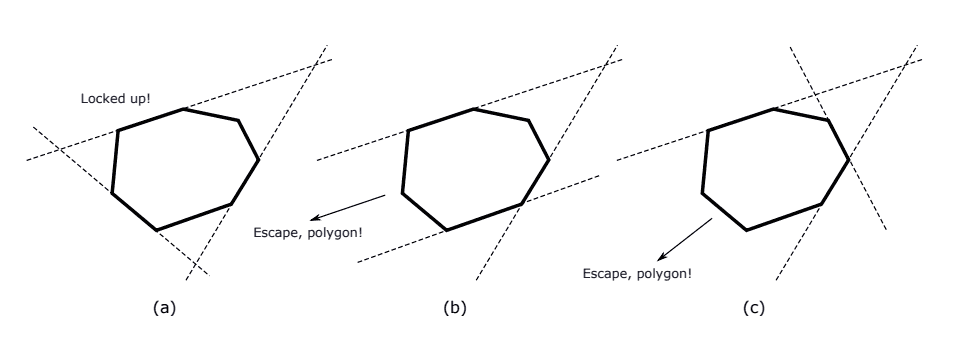### E - Escape, Polygon!

##### Languages: MS C# .NET 4.7.2053,GNU G++11 5.1.0 ...

A suspicious-looking convex polygon wants to escape its current position by translating itself along some straight-line direction. Three very diligent straight lines want to lock it up by placing themselves along three distinct sides of the polygon. Then, if the triple of lines defines a triangle and the polygon lies inside this triangle, it will be locked up. Otherwise, it will escape.Figure (a) above illustrates a triple that will lock the polygon up. For (b), the lines do not define a triangle since two of them are parallel, and so the polygon will escape. In (c), the polygon lies outside the triangle defined by the triple and it will easily escape.

Given a polygon, you must compute the number of distinct triples of lines that can lock the polygon up.

#### Input

The first line contains an integer $N$ ($3\leq N\leq 10^5$) representing the number of vertices of the polygon. Each of the next $N$ lines describes a vertex with two integers $X$ and $Y$ ($-10^8\leq X,Y\leq 10^8$) indicating the coordinates of the vertex in the $XY$ plane. The vertices are given in counter-clockwise order and they define a simple convex polygon. No three vertices are collinear.

#### Output

Output a single line with an integer indicating the number of distinct triples of lines that can lock the given polygon up.

#### Sample test(s)

Input
4 0 0 10 0 10 10 0 5
Output
1
Input
8 0 32 -12 15 -10 -10 0 -12 10 -12 22 0 25 10 18 20
Output
18
Input
3 10 -10 0 10 -10 -10
Output
1
Input
6 -100000000 131 -50000067 -100000000 50000014 -100000000 100000000 -109 70000173 100000000 -90000011 100000000
Output
6
Input
4 0 0 10 0 10 10 0 10
Output
0